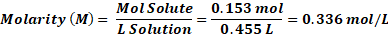It looks like you're using Internet Explorer 11 or older. This website works best with modern browsers such as the latest versions of Chrome, Firefox, Safari, and Edge. If you continue with this browser, you may see unexpected results.

# Chemistry

Properties of Solutions

Solutions are homogeneous mixtures of two or more substances. The substance with the highest concentration within the mixture is known as a solvent, and the other substance(s) are called the solute(s). Solutions have uniform composition where solvent and solute cannot be seen separately with the naked eye.

A solution can be in any of the three states of matter: gas, solid, or liquid. For example, when sugar (C12H22O11), a covalent solid, is dissolved in water, its molecules get uniformly dispersed in water molecules and form a homogeneous aqueous solution, as shown below. The subscript “aq” beside C12 H22 O11 shows that sugar molecules are solutes and are evenly distributed in the aqueous solution where water is the solvent.

C12 H22 O11(s) C12 H22 O11(aq)

When ionic compounds are dissolved in water, they dissociate to yield ions in an aqueous solution. For example, when potassium dichromate (K2Cr2O7) dissolves in water, it dissociates to give potassium ions and dichromate ions that are uniformly dispersed in the mixture, as shown below:

K2Cr2O7(s) 2K+(aq) + Cr2O72−(aq)

Solutions display the following defining characteristics:

• Homogeneous: once the solute and solvent are mixed, the mixture has the same composition at all points.
• The solution has the same physical state as the solvent. For example, when sugar (solid solute) is dissolved in water (liquid solvent), the final product is an aqueous solution in a liquid state.
• Dissolved solute does not settle at the bottom.
• In a solution, the solute molecules, atoms, or ions are closely surrounded by solvent particles.
• The amount of solute that can be dissolved in a solvent is determined by the solubility (discussed further in the next section).

Concentration and Molarity

• The concentration of a solution measures the amount of solute that is dissolved in a particular amount of solvent. Concentration is measured using different units, some of which are explained below.
• Solubility in a specific solvent refers to the maximum concentration that can be obtained under given conditions.
• If the solubility of a solution is equal to its concentration, the solution is saturated with the solute. If the solute's concentration is lower than the solubility, the solution is considered unsaturated. On the other hand, a supersaturated solution is one whose solute concentration is higher than its solubility.
• A solution with a low concentration of solute is known as a dilute solution, whereas one with a high concentration is called a concentrated solution.

Concentration as a Percent

Concentration can be expressed as a mass percent when the mass of the solute and solution are known. It can be calculated using the following formula: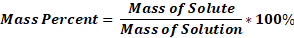Example:

In the given solution, there is 110.605 g of solvent and 6.0508 g of solute present. Find the mass percent.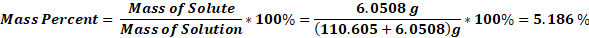Concentration as PPM

Parts per million (ppm) is defined as the ratio of solute-to-solution mass multiplied by 106, as shown below: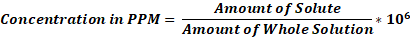ppm = 1 g/m3 = 1 mg/ L= 1 μg/mL

Example:

A solution has a concentration of 1.35 g /L. What is its concentration in ppm?

Convert the mass in grams to a mass in milligrams:

1.35 g = 1.35 × 1000 mg = 1350 mg

Re-write the concentration in mg/L:

Concentration in mg/L= 1350 mg/ L = 1350 ppm

Molarity

Molarity, or molar concentration of a solution, is the amount of solute (in moles) per litre of solution. It is calculated using the following formula: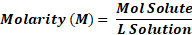Example:

A 455-mL soft drink sample contains 0.153 mol of sucrose (table sugar). What is the molar concentration of sucrose in the beverage?

Converting 455 ml to L gives 0.455 L